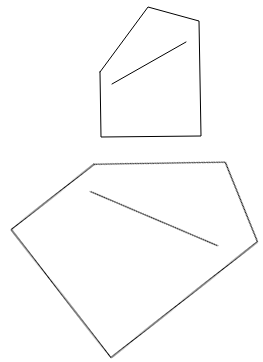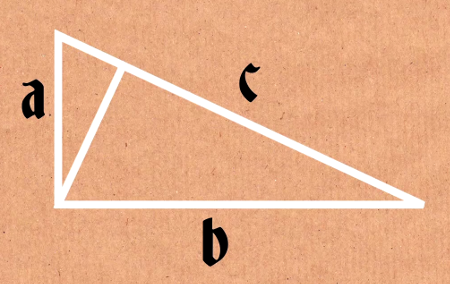The Numberphile video below is quite excellent, but some viewers might appreciate clarification on the topic. Watching the video is not required to follow this post.

## The Motivation

We wish to prove the Pythagorean theorem using scale and similarity as our foundation, rather than the properties of squares and triangles together. In fact, we will hardly use square shapes at all, though square angles and squared numbers will be prominent. A strong benefit to this similarity approach is that it only requires one very simple drawing, which is a huge asset compared to most other proofs.[^n] Also, this method of proof tells us something deeper about the theorem, that there is a more general statement that can be made, which we call the "blob Pythagorean theorem" here.

## The Setup

We assume a (property of similar figures)(prop-of-sim-figs) that relates their linear and area measurements.

(start prop-of-sim-figs)

Property. Given two similar figures, if you draw a line in the first and scale it to get an (appropriately scaled)(app-scaled) line in the second, the linear ratio between the lengths of the lines is independent of what line you chose to draw. Further, if you obtain an area ratio (in the same way,)(prop-sim-same-way) the result will be the square of the linear ratio.

(start app-scaled)

By "appropriately scaled", I mean what is shown in the diagram below.Take the first figure and the line you drew in it, and scale them together until the scaled figure is congruent to the second figure. Now the scaled line is "appropriately scaled".

(stop app-scaled)

(start prop-sim-same-way)

By "in the same way", I mean that you measure the ratio between the area of any shape you draw and the area of its appropriately scaled version.

(stop prop-sim-same-way)

(stop prop-of-sim-figs)

We will also need a (theorem to identify similar figures)(thm-ident-sim-fig), that is, to prove that two triangles we suspect are similar actually are.

(start thm-ident-sim-fig)

Theorem. By definition, two triangles are similar if and only if their three corresponding pairs of angles are equal. Since the angles of each triangle must sum to 180°, it is equivalent to say that two of the three corresponding pairs of angles are equal. (Why?)(sum-to-180)

(start sum-to-180)

If two pairs of angles measure $$x$$ and $$y$$, then the third angle on each triangle must measure $$180 − x − y$$, so the third pair must be equal. The converse is trivial: three equal pairs implies two pairs are equal.

(stop sum-to-180)

(stop thm-ident-sim-fig)

Note. These results are also discussed on Wikipedia and Khan Academy, as well as many others.

We (generalize)(generalize) the (Pythagorean theorem)(pyth-thm) to become a set of (blob Pythagorean theorems)(blob-pyth-thm), none of which we assume to be true. <!TODO: insert video timestamps here>

(start generalize)

By "generalize", I mean that the original Pythagorean theorem is a special case of the more general blob Pythagorean theorem. The special case is when you choose the blob to be a square, which matches the geometric description of the original theorem.

(stop generalize)

(start pyth-thm)

Original Theorem. Given a right triangle with [small sides][(the legs)] of lengths $$a$$ and $$b$$, and with a [long side][(the hypotenuse)] of length $$c$$, it must be true that $$a^2 + b^2 = c^2$$. This is the algebraic description; geometrically, if you draw three squares, each one sharing a side with one of the triangle's sides, then the sum of the two smaller areas equals the area of the largest.

(stop pyth-thm)

(start blob-pyth-thm)

Blob Theorem. Given a right triangle and a figure with nonzero area (called a blob), we may draw a line somewhere on the blob. If we scale the blob-and-line three times, each time scaling the line to be as long as one of the sides of the right triangle, then the sum of the two smaller blob areas equals the area of the largest blob.

(stop blob-pyth-thm)

## The Proof

The property of similarity shows that (all blob Pythagorean theorems are equivalent)(all-blob-equiv). (How?)(all-blob-equiv-how)

(start all-blob-equiv)

That is, knowing the blob Pythagorean theorem holds for one blob implies it is true for any other blob.

(stop all-blob-equiv)

(start all-blob-equiv-how)

Suppose that we have found a figure, called Blobby with nonzero area $$B$$, and line, called Liney with length $$l$$, for which the blob Pythagorean theorem holds on a particular right triangle with lengths $$a, b, c$$, with $$c$$ greatest. When we scale Blobby-and-Liney three times, the linear ratios for the scalings are $$\frac{a}{l}, \frac{b}{l}, \frac{c}{l}$$. (Why?)(linear-ratios-why)

(start linear-ratios-why)

The first time we scale Blobby-and-Liney, we scale Liney from a length $$l$$ to a length $$a$$, giving a linear ratio of $$\frac{a}{l}$$. Similarly, the other two scalings have linear ratios of $$\frac{b}{l}$$ and $$\frac{c}{l}$$.

(stop linear-ratios-why)

With those linear ratios, the property of similarity implies that the scaled versions of Blobby must have areas $$\newcommand\pfrac{\left(\frac{#1}{#2}\right)}\pfrac{a}{l}^2B, \pfrac{b}{l}^2B,\pfrac{c}{l}^2B$$. The blob Pythagorean theorem for Blobby says that the sum of the first two is equal to the last, which is equivalent to saying that $$a^2 + b^2 = c^2$$. (Why?)(equiv-to-orig-why)

(start equiv-to-orig-why)

First note that reordering the multiplication in the first equation can simplify it to having a common factor in every term:

$\pfrac{a}{l}^2B + \pfrac{b}{l}^2B = \pfrac{c}{l}^2B \\ \pfrac{a^2}{l^2}B + \pfrac{b^2}{l^2}B = \pfrac{c^2}{l^2}B \\ a^2\pfrac{B}{l^2} + b^2\pfrac{B}{l^2} = c^2\pfrac{B}{l^2}$

Then, given either that equation or $$a^2 + b^2 = c^2$$ to start with, we may obtain the other, because we can multiply and divide by $$B/l^2$$ freely and maintain equality. (This is what requires Blobby to have nonzero area. Otherwise, dividing by $$B$$ would be a problem.)

(stop equiv-to-orig-why)

Since every blob Pythagorean theorem on a particular triangle is equivalent to $$a^2 + b^2 = c^2$$, they are all equivalent to each other.

(stop all-blob-equiv-how)

The theorem to identify similar triangles shows that when the blob is the right triangle you are given, the blob Pythagorean theorem is true. (How?)(blob-right-tri-how)

(start blob-right-tri-how)

Let's show that one particular blob Pythagorean theorem is true. We are given a right triangle in the theorem; let this be our blob. For the choice of line, choose the hypotenuse. The magic step comes in noticing that there is a clever way to cut our blob that defines all our scaled blobs at once: (taken from the video)This line is very carefully drawn to be the one coming from the right angle and meeting the opposite side at right angles. Thus, we now have three triangles to consider, and each one shares its hypotenuse with a side of the original; if they are all similar triangles, then these are in fact the blobs we want, and it is clear that the two smaller areas add up to the larger, since we cut up the larger to get the two smaller ones.

To see that the triangles are similar, we use the theorem to identify similar triangles. Each one has a right angle, so we know they have one angle in common already. For the upper-left small triangle, it shares the upper-left angle with the original, and thus must be similar to it. For the bottom-right small triangle, it shares the bottom-right angle with the original, and thus must be similar to it.

With that, we are done. The triangles are all similar, scaled appropriately to be the scaled blobs. The right-triangled-blob Pythagorean theorem must be true, so all blob Pythagorean theorems must be true.

(stop blob-right-tri-how)

Together, these facts imply the blob Pythagorean theorem is true for all blobs, including that of the square blob, which is the original Pythagorean Theorem.